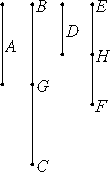# Proposition 9

If a number is a part of a number, and another is the same part of another, then alternately, whatever part or parts the first is of the third, the same part, or the same parts, the second is of the fourth.

Let the number A be a part of the number BC, and and another number D be the same part of another number EF that A is of BC.

I say that, alternately, BC is the same part or parts of EF that A is of D.Since D is the same part of EF that A is of BC, therefore there are as many numbers BC equal to A as there are also in EF equal to D.

Divide BC into the numbers equal to A, namely BG and GC, and divide EF into those equal to D, namely EH and HF. Then the multitude of BG and GC equals the multitude of EH and HF.

Now, since the numbers BG and GC equal one another, and the numbers EH and HF also equal one another, while the multitude of BG and GC equals the multitude of EH and HF, therefore GC is the same part or parts of HF that BG is of EH, so that, in addition, the sum BC is the same part or parts of the sum EF that BG is of EH.

But BG equals A, and EH equals D, therefore BC is the same part or parts of EF that A is of D.

Therefore, if a number is a part of a number, and another is the same part of another, then alternately, whatever part of parts the first is of the third, the same part, or the same parts, the second is of the fourth.

Q.E.D.

## Guide

In this proposition, Euclid shows that if a = b/n, and d = e/n, and if a = (p/q)d, then b = (p/q)e.

The sample value taken for 1/n in the proof is 1/2.

Proposition VII.15 can be construed as a special case of this one.

This proposition is used in the proof of the next.